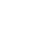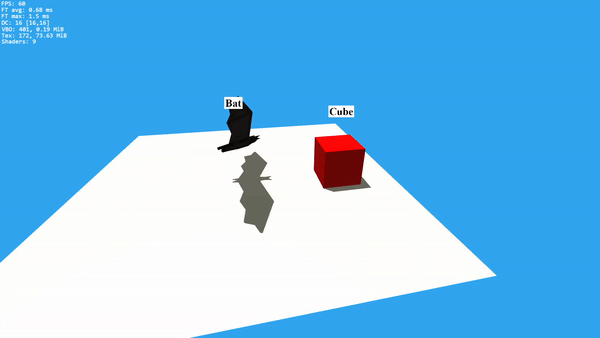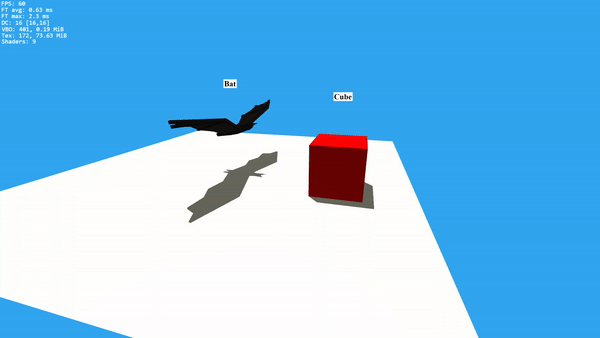pingpoli.de

# Sparrow WebGL Devlog 14: Billboards

## How to implement billboards in WebGL and JavaScript

14.11.2023 - 17:01
Imagine an NPC in a video game. You can see their name above their head. Now move a few steps and look at the NPC again. You can still see and read their name. Just like Mona Lisa’s eyes, the text always faces you no matter where you go. This technique is known as billboards in computer graphics.

Rendering text that always faces the camera is one of many situations where billboards are useful. Other common uses are health bars and 3D particle systems. Fire is typically just a bunch of animated particles that face the camera. Finally, there is another trick that graphics programmers use to save performance in large and open environments. Textured quads that are always rotated toward the player can replace 3D models in the distance. This would be obvious nearby, but it’s almost undetectable in the distance.

# Billboards in WebGL

What does it mean when a quad always has to face the camera? It has to be rotated the same way as the camera and be parallel to the camera plane. We could do this with two rotation matrices to copy the yaw and pitch of the camera and combine them into the model matrix of the quad, but this isn’t the best approach.

There are many different ways you can implement billboards. With OpenGL, you can use geometry shaders to generate the vertices of the quad so that they face the camera. WebGL, however, doesn’t support geometry shaders, so let’s use a different solution instead.

First, a quick reminder about world space and camera space in WebGL. World space describes the default coordinate system of the scene. For example, a cube is at some coordinates like (2,3,-4) and the camera is somewhere looking in a random direction. The projection matrix, however, expects the camera to be at (0,0,0) facing the negative z-axis, which is also known as the camera space. The view matrix is used to convert from world space to camera space.

In camera space, the up vector is (0,1,0), and the right vector is (1,0,0). All we need to know is what these vectors are in world space and we can easily create a quad that’s parallel to the camera plane. Mathematically, this is done with the inverse view matrix, but conveniently, we can get the values we need directly from the normal view matrix.

```// view matrix uniform mat4 u_M_v; [...] vec3 camera_right = vec3( u_M_v , u_M_v , u_M_v ); vec3 camera_up = vec3( u_M_v , u_M_v , u_M_v ); ```

With these two vectors, we can calculate the position of the vertices of the billboarded quad. For this, we need the world position of the center of the quad, the offsets of the four quad corners, and the size of the billboard. All combined into the full vertex shader, we get this:

```#version 300 es // offsets of the four quad vertices layout ( location = 0 ) in vec2 vertexPosition; layout ( location = 2 ) in vec2 vertexUV; // view matrix uniform mat4 u_M_v; // view projection matrix uniform mat4 u_M_vp; // world position of the quad center uniform vec3 u_position; // size of the quad uniform vec2 u_size; out vec2 uv; void main() { vec3 camera_right = vec3( u_M_v , u_M_v , u_M_v ); vec3 camera_up = vec3( u_M_v , u_M_v , u_M_v ); // calculate the vertex positions of the quad in world space vec3 vertexPosition_worldspace = u_position + camera_right * vertexPosition.x * u_size.x + camera_up * vertexPosition.y * u_size.y; gl_Position = u_M_vp * vec4( vertexPosition_worldspace , 1.0 ); uv = vertexUV; } ```

The vertices of the quad are just simple 2D offsets from -0.5 to 0.5. In the shader, this is multiplied by the uniform size value which controls the actual size of the billboarded quad. Here is how to set up the quad vertices and their uvs:

```var vertices = new Float32Array( [ -0.5 , -0.5 , -0.5 , 0.5 , 0.5 , -0.5 , 0.5 , 0.5 ] ); vertexBuffer.bind(); vertexBuffer.bufferData( vertices , gl.STATIC_DRAW ); var uvs = new Float32Array( [ 0 , 1 , 0 , 0 , 1 , 1 , 1 , 0 ] ); uvBuffer.bind(); uvBuffer.bufferData( uvs , gl.STATIC_DRAW ); ```

The billboard always faces the camera, but it changes size when the camera zooms:When using the approach above, the billboards will behave like normal objects in the scene, i.e. when you move further away, the billboard will be smaller. This is the expected behavior for particles or billboarded objects in the distance. However, for something like names or HP bars, it would be preferred if the billboard always stayed the same size, so the text remains readable.

# Fixed-size billboards

When you want fixed-size billboards the code is even simpler, because the quad can be generated after the projection, which will be in screen space. However, we need the size of the window or screen as an additional uniform, so the quad always stays the same size and doesn’t stretch when the window is resized.

```// project the position of the quad center gl_Position = u_M_vp * vec4( u_position , 1.0 ); // perspective division gl_Position /= gl_Position.w; // apply the offsets for the corners gl_Position.xy += vertexPosition.xy * vec2( u_size.x/u_windowSize.x , u_size.y/u_windowSize.y ); ```

The billboard always stays the same size and the text remains readable:# Sparrow

This is still a devlog about creating my Sparrow WebGL engine, but I wanted to try to write this one more like a tutorial to see how it does. Billboards are small enough in scope that I can go over most of the code. The implementation in the engine is a bit more complicated because it has a billboard manager, different types of billboards, and additional options. The main type I need is text billboards to label certain elements of a scene. Like most engine features, this is only a starting point and I will add more features when I need them.

Besides adding the billboards, I also fixed some bugs in the path-finding code, like zero-length paths crashing the engine. Finally, I worked a lot on path-smoothing so the returned path actually makes sense. It’s still not guaranteed to be the mathematically shortest path, but it’s convincing enough for now.

by Christian - 14.11.2023 - 17:01